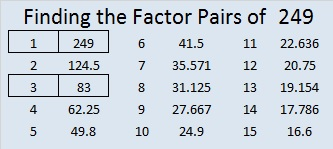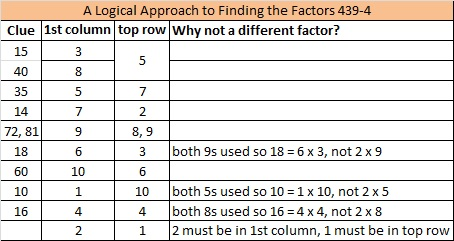# 249 and Level 4

• 249 is a composite number.
• Prime factorization: 249 = 3 x 83
• The exponents in the prime factorization are 1, and 1. Adding one to each and multiplying we get (1 + 1)(1 + 1) = 2 x 2 = 4. Therefore 249 has 4 factors.
• Factors of 249: 1, 3, 83, 249
• Factor pairs: 249 = 1 x 249 or 3 x 83
• 249 has no square factors so its square root cannot be simplified. √249 ≈ 15.7797Print the puzzles or type the factors on this excel file: 10 Factors 2014-09-29This site uses Akismet to reduce spam. Learn how your comment data is processed.